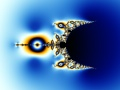# Nova (Julia, Mandelbrot)The Nova fractal is a modified Newton fractal. The Julia version can be used as a normal Newton fractal, but there are all kinds of other possibilities with intriguing spirals.

Both Mandelbrot and Julia versions of the Nova fractal are available as fractal formulas in Standard.ufm and as fractal formula plug-ins in Standard.ulb.

Use the Nova (Mandelbrot) formula to switch to interesting Nova (Julia) sets. The standard Newton fractals can be found in the empty circle to the right.

The formulas provide the following parameters:

 Start Value (Mandelbrot only) For well-formed fractals, this should be set to (1, 0). Other values create distorted shapes that can be interesting, but they are usually not as well-formed. Julia Seed (Julia only) This parameter specifies the point in the Mandelbrot version that corresponds to the current Julia version. It defines the shape and behavior of the fractal. Use the Switch feature to select good values. Exponent Increase the real value of the exponent to create more complex fractals. Non-integer real values and non-zero imaginary values create irregular fractals. Bailout Specifies the magnitude of z at which the formula will stop iterating. Since z converges to a fixed value, smaller values will give more detailed images. Relaxation This can be used to influence the convergence of the fractal. Changing this parameter will twist and transform the fractal.